Skip to main content

Thank you for visiting nature.com. You are using a browser version with limited support for CSS. To obtain the best experience, we recommend you use a more up to date browser (or turn off compatibility mode in Internet Explorer). In the meantime, to ensure continued support, we are displaying the site without styles and JavaScript.

# Phonon arithmetic in a trapped ion system

## Abstract

Single-quantum level operations are important tools to manipulate a quantum state. Annihilation or creation of single particles translates a quantum state to another by adding or subtracting a particle, depending on how many are already in the given state. The operations are probabilistic and the success rate has yet been low in their experimental realization. Here we experimentally demonstrate (near) deterministic addition and subtraction of a bosonic particle, in particular a phonon of ionic motion in a harmonic potential. We realize the operations by coupling phonons to an auxiliary two-level system and applying transitionless adiabatic passage. We show handy repetition of the operations on various initial states and demonstrate by the reconstruction of the density matrices that the operations preserve coherences. We observe the transformation of a classical state to a highly non-classical one and a Gaussian state to a non-Gaussian one by applying a sequence of operations deterministically.

## Introduction

In quantum mechanics, bosonic creation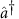and annihilation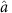operators bear the following operator relations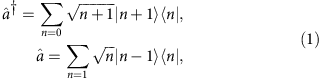where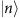stands for a Fock state of n bosons. The proportionality factors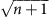and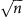appear due to the symmetric indistinguishable nature of bosons1,2. Thus, the addition or subtraction in quantum domain involves the modification of the probability amplitude of state due to the excitation n-dependent factor. The bosonic annihilation and creation operation have been proposed to be a building block to generate an arbitrary quantum state3,4, to distill entanglement or non-locality5 and to transform a classical state to a non-classical state6. In recent times, there have been seminal works to realize the bosonic operations at the single-boson level for the test of foundations and applications of quantum mechanics7,8,9,10,11,12,13. However, such bosonic operations are not trace preserving and inherently probabilistic. The probability of success has so far been extremely low in their implementation for photonic fields. A higher probability may be obtained at the expense of lowering the performance fidelity14. Owing to this, a repetition of these operations has been limited.

The conventional addition and subtraction of a particle can be written as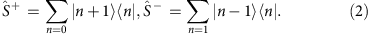The addition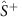takes the n particle state to the (n+1) state, whereas the subtraction operationbrings the n state to the (n−1) state without incurring additional factors. Seeing the form of the operations in equation (2), we immediately recognize thatis a deterministic process while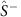may not be, as it is not possible to subtract a particle from vacuum |0〉. When the vacuum component of the initial state is small, the subtraction can be done near-deterministically. In recent times, there have been theoretical proposals of the operations (equation (2)) for the generation of an arbitrary quantum state15, the measurement of vacuum16, the transformation to a non-classical state17 and the amplification of a quantum state18. In particular, such arithmetic operations form an important component of a qubit gate operation for ions in a harmonic potential19. The operations (equation (2)) were also suggested as the elements of a phase operator20. Beyond the quantum-state engineering, the subtraction can be used for the sub-Doppler cooling in the trapped ion system21.

In this study, we experimentally demonstrate deterministic addition and near-deterministic subtraction of a bosonic particle, in particular a phonon of a 171Yb+ ion trapped in a harmonic potential. We realize the operations by coupling phonons to an auxiliary two-level system, so called the hybrid scheme of discrete and continuous variable22, and applying a transitionless quantum-driving scheme. We perform the operations on superpositions of Fock states and coherent states, and we demonstrate that our single-phonon operations are (near) deterministic and preserve coherence. By applying a sequence of operations deterministically, we show that classical states are turned into non-classical ones manifesting highly sub-Poissonian statistics and negativity in the Wigner function.

## Results

### Experimental implementations

We implement theandoperations of equation (2) on a vibrational mode of frequency ωX for a single trapped 171Yb+ ion in a three-dimensional harmonic potential23 through its interaction with the two-level system of atomic energy levels. The harmonic potential is generated by an oscillating electric field in the radial axis with trap frequency ωX=(2π)2.8 MHz. The two-level system is represented by two hyperfine states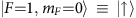and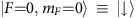of the S1/2 manifold with the transition frequency ωHF=(2π)12.6428 GHz. As shown in Fig. 1a, the anti-Jaynes–Cummings (aJC) interaction or blue-sideband transition,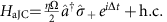, is realized by the stimulated Raman laser beams with beat-note frequency (ωR1ωR2)=(ωHF+ωX)+Δ. Here, Ω is the Rabi frequency of the two-level system,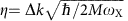the Lamb–Dicke parameter, Δk the net wave vector of the Raman laser beams and M the mass of 171Yb+ ion. The aJC coupling produces the transition between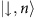and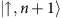with the oscillation frequency of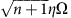, where thefactor comes from the fundamental property of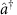andoperators in equation (1). Therefore, the application of the simple aJC interaction does not transfertoin an n-independent manner at a fixed duration of time.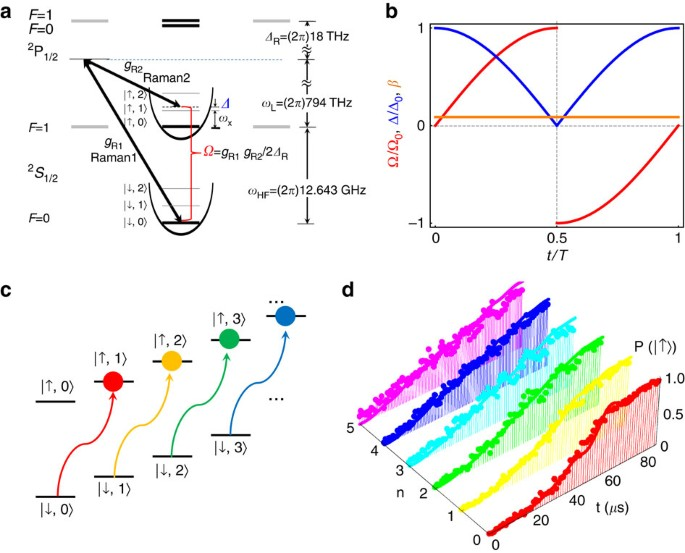Figure 1: Experimental scheme and dynamic and adiabatic transition by anti-Jaynes–Cummings interaction.

The full population transfer independent of the initial motion state, the uniform blue-sideband transition,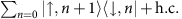, can be obtained by the application of the stimulated Raman adiabatic passage24,25,26. The adiabatic scheme provides a robust transfer against the variations in the transition rate either from the intensity change of the control Raman beams or from the property of the transitions25. Therefore, a properly designed adiabatic passage would allow a decent state transfer for a wide range of phonon number states through the aJC interaction, despite thedependence. In the adiabatic passage, typically ηΩ slowly increases at the beginning and decreases at the end, that is, Ω(t)=Ω0 sin(πt/T), whereas the detuning Δ changes according to Δ(t)=Δ0 cos(πt/T), where T is the total transfer time, across the resonance. However, for the applicability of the scheme to a wide range of initial phonon numbers with high fidelity, we should set Δ0 as high as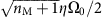, where nM is the largest phonon number for the transfer and should fulfill the adiabatic condition,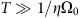. In our experimental conditions, the reasonable duration T for such an adiabatic transfer is around 21 times that of π-pulse duration for the blue-sideband transition of the ground state π/ηΩ0, when we include up to the maximum phonon number nM=6.

In recent times, the transitionless quantum-driving scheme27,28,29,30 has been developed to speed up the adiabatic control. When the transfer is non-adiabatic, it introduces an additional term in the Hamiltonian of the instantaneous basis, which is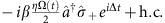, where β is in the order of π/ηΩ0/2T, the ratio between the π-pulse duration and the total operation time. By adding a counter-diabatic term in the control, we can suppress the non-adiabatic excitation with a reasonable speed up over the adiabatic passage. For the aJC interaction, the optimal values of Δ0 and β are dependent on the phonon number n for the given Ω0. In our experiment, we optimize Δ0 and β for the case of the geometric average of the minimum and maximum phonon number,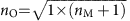. By doing this, we are able to reduce the total duration of the operation from 21 to 7 times π/ηΩ0 without sacrificing the fidelity of the rapid adiabatic passage for the same range of phonons nM=6.

To rigorously achieve the uniform blue-sideband operation, it is important not only to increase the phonon number but also to preserve the relative phases between component states of the quantum state. For our previous realization23, the different extra phases were accumulated depending on the phonon number of the initial state, which prevented from keeping the initial phase coherences (see ‘AC Stark shift compensation’ in Methods). In this study, we have developed a sequence of operations compensating the phonon-number-dependent phases based on the spin-echo principle. As shown in Fig. 1b, we invert the sign of Ω and reverse the control of Δ in the middle of the sequence of the operation, which symmetrizes the whole operation and produces the accumulated phases of opposite signs before and after the inversion and reverse. Therefore, the total phases are cancelled out at the end of the operation (see ‘AC Stark shift compensation’ in Methods).

### Conventional phase-coherent addition

We implement the coherent addition operationin equation (2) by applying the uniform blue-sideband transfer followed by the π-pulse of carrier transition as shown in Fig. 2a. By this operation, we add single phonons deterministically, independent of the initial number of phonons. To manifest the coherence property of the addition operations, we prepare an initial state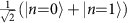, apply the operations up to three times and measure the density matrices of the resulting phonon states. As shown in Fig. 2b, the coherences represented by the off-diagonal terms of the density matrix clearly remain after the multiple addition processes up to three times.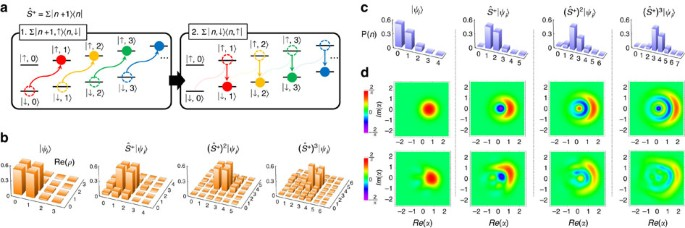Figure 2: Schematic diagram and experimental results for the phonon addition.

We also consider an initial coherent state of the amplitude α=0.81, which is achieved by displacing the vacuum. The density matrix is reconstructed (see Fig. 2c,d) by using the iterative maximum-likelihood algorithm31 on the phonon number distributions for eight different angles (see ‘Reconstruction of the phonon density matrix’ in Methods). The phonon number distributions are obtained by observing the time evolutions of the standard blue-sideband transitions, similar to the direct reconstruction scheme of the phonon density matrix32. One immediate consequence of adding single phonons on the coherent state is the production of sub-Poissonian phonon statistics, because the addition increases the average phonon number but not the shape of the distribution nor the variance. We observe that the ratios between the variance and the average phonon number reduce from 1 to 0.43, 0.39 and 0.2, after 1–3 single-phonon additions, respectively. Applying the first addition operation, we detect negativity in the Wigner function as shown in the second column of Fig. 2d. It is important to note that the addition operation, which converts a coherent state to a highly non-Gaussian and non-classical state, is deterministic unlike the case ofoperation9,10,11. There is a technical limit in the number of additions we can apply, owing to the validity of the adiabatic approximation and the heating process of phonons33. Under this limitation, we could perform the operations three times without the significant loss of fidelity. As shown in Fig. 2d, the experimental results and the theoretical predictions for the Wigner functions are in agreement, except the shift of the orientation, which would come from the phase offset between the preparation and the analysing pulses. This is significant in comparison with the photonic realization of bosonic operations of single-photon creation and annihilation11. We detect negativity in the Wigner function as shown in the second column of Fig. 2, where we obtain the Wigner function of the state from the reconstructed density matrix.

### Conventional subtraction operation

The subtraction operationin equation (2) is realized by reversing the sequence of the addition operation, that is, the application of the π-pulse of carrier transition and the uniform blue-sideband transfer, followed by the fluorescence detection as shown in Fig. 3a. The zero phonon state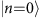is eliminated after the subtraction, which is implemented by the conditional measurement in our experimental scheme. After the detection sequence, we only collect the data with no fluorescence, which has the success rate given by the probability of the non-zero phonon states. We examine the performance of the subtraction operation with an initial phonon superposition state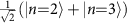. As shown in Fig. 3b, the subtraction operation reduces the phonon excitation by one quanta. After the second application of the subtraction, the off-diagonal terms of the density matrix are significantly reduced, which shows the current limit in experiments due to heating of the system. We also prepare a coherent state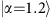and apply the subtraction twice as shown in Fig. 3c, which shows qualitatively that the subtraction operation works for any initial quantum state. The subtraction operation can squeeze a coherent state, which is different from annihilationthat has the coherence state as its eigenstate. However, our experimental precision is not high enough to observe the squeezing effect.Figure 3: Schematic diagram and experimental results of phonon subtraction.

### Commutation relation of addition and subtraction operations

We study experimentally how the quantum states are changed depending on the order of the addition and subtraction for an initial coherent state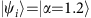. If we add and then subtract, the state after the sequence is the same as the original one, as there is no amplitude modification. For the case of subtraction-then-addition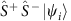the final state does not have vacuum component, because a vacuum component is annihilated byand cannot be restored by. Figure 4b shows the experimental result of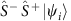, which is basically identical to the initial state of Fig. 4a. Figure 4c shows the result after the operation of, where there is no significant vacuum component in the density matrix. The vacuum component is not perfectly absent because of the detection error during the projective measurement based on the atomic fluorescence and heating of the system. The fluorescence detection duration is comparable to the motional coherence time of our system, which makes the off-diagonal part of the final state suppressed significantly (see ‘Error analysis’ in Methods). Our experimental result is well in line with the non-commuting relation of the Susskind–Glogower’s phase operators, that is,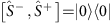(ref. 20).Figure 4: Experimental results after addition-then-subtraction and subtraction-then-addition.

## Discussion

Owing to the capability of deterministically generating a non-classical and non-Gaussian states, the conventional addition and subtraction operations provide an efficient scheme for quantum state engineering34,35. The current work can also be a stepping stone to realize the ion qubit gate operation as proposed by Schneider et al.19 without having to worry about long operation times and loss of coherences. This scheme may be further applied to various quantum optics setups such as cavity quantum electrodynamics and optomechanics. We note a theoretical scheme that the non-Gaussian information of a phonon state would be transferred to that of photon through phonon–photon coupling36,37. It has also been discussed that the conventional arithmetic addition and subtraction can be used to measure the vacuum state without disturbing the state16 and therefore it can be directly applied to construct the Q-function of a quantum state of motion. We have noted that an arithmetic subtraction was performed for a photonic system38.

## Methods

### AC Stark shift compensation

The AC Stark shift in the adiabatic operations mainly comes from the off-resonant coupling to the carrier transition, the transition between S1/2P1/2 states of 171Yb+ ion and the other radial motional mode (ωYωX+(2π)0.4 MHz). The dominant AC stark shift comes from the carrier transition of frequency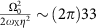kHz with Ω0=(2π)38.5 kHz and the Lamb–Dicke parameter η=0.089. The amount of the shift brought by the Y mode is given by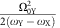, that is, 20 times smaller than that from the carrier coupling. The AC stark shift between qubit states from the Raman laser beams due to S1/2P1/2 transition is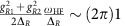kHz, where gR1 and gR2 are the coupling strengths of Raman 1 and Raman 2 beams, respectively, and the ΔR=(2π) 18 THz is the detuning from the level of 2P1/2.

We consider total AC stark shift as the form of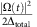, where Δtotal is the detuning effectively including all the possible origin of AC stark shifts discussed above. We obtain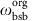and Δtotal by fitting the several points of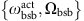with the equation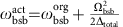. The actual frequency of blue-sideband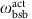is measured by observing the resonant excitation. Including the AC stark shift, the actual waveform of Ω(t) that we apply on our arbitrary waveform generator is as follows: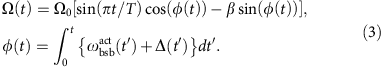Here, Δ(t′)=Δ0 cos(πt/T) and we note that the imaginary part in original form of Ω(t)=Ω0[sin(πt/T)+] is changed to sin-wave, which has the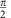phase difference. Here, φ(t) is calculated as follows: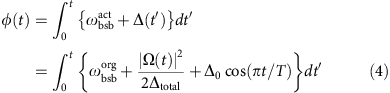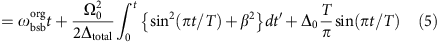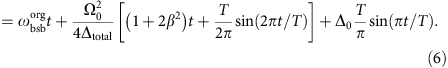### Reconstruction of the phonon density matrix

We use an iterative algorithm in ref. 31 for the reconstruction of a quantum state. It consists of a maximum-likelihood estimation solved by the expectation-maximization algorithm followed by a unitary transformation of the eigenbasis of the density matrix ρ.

Based on the measured phonon distribution fn by N measurements after a displacement of the quantum state, we aim to get real probabilities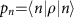that are as close to the observed frequencies fn as possible, subject to the maximum-likelihood functional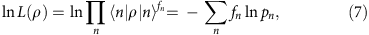from which we reconstruct ρ. This likelihood functional can be interpreted as a linear and positive problem in the classical signal processing: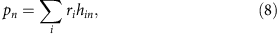where ri is the eigenvalues of ρ and hin is a positive kernel. We can solve this linear and positive problem with the expectation-maximization algorithm39,40: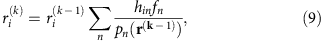which is initially set to a positive vector r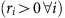. This is repeated for different displacements.

The second part of the reconstruction scheme aims at getting the eigenbasis diagonalizing the density matrix. This part consists of two steps: reconstruction of the eigenvectors of ρ in a fixed basis and rotation of the basis using a unitary transformation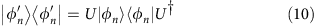with the infinitesimal form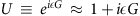and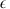is a small positive real number. G=i[ρ, R] is chosen as a Hermitian generator of the unitary transformation, where R is a semipositive definite Hermitian operator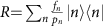.

Starting from some positive initial density matrix ρ, we continue repetition of first finding eigenvalues ri using the expectation-maximization iterative algorithm (equation (9)) and then finding eigenvectors φi by unitarily transforming the old ones. The likelihood of the estimate pn is increased and we finally reach to determine the density matrix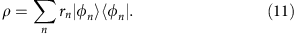### Error analysis

Dominant error comes from the phonon heating process caused by the electric-field noise from the trapped electrodes41. Heating decreases the Fock state preparation fidelity and affects the adiabatic blue sideband process. Its time evolution is known to be described by41,42: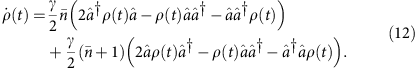where γ is the coupling strength between the ion motion and the thermal reservoir and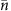is the average phonon number for the thermal reservoir. In our experimental setup, the heating rate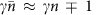is 150 Hz. It can be reduced by using a large trap, cleaning the electrodes43 (equivalent to reducing γ) or cooling the trap (equivalent to reducing) (ref. 44).

Error may be caused by fitting, as some noise in the blue-sideband curves may be recognized as a high phonon population. Although the Fock state preparation error will be involved in the transition probability, fortunately the error for a small n is relatively insignificant and the population mainly resides in small n-values for the initial states we prepared in the experiments.

There are some other small errors that we did not calculate or simulate, including the fluctuation of the trap frequency 0.1 kHz coming from 2% intensity fluctuation of Raman laser and 1 kHz trap frequency fluctuation coming from sudden changes of the ion position. We see how much our experimental results are modified when comparing with the simulations23.

## Additional information

How to cite this article: Um, M. et al. Phonon arithmetic in a trapped ion system. Nat. Commun. 7:11410 doi: 10.1038/ncomms11410 (2016).

## References

1. 1

Bose, S. N. Plancks gesetz und lichtquantenhypothese. Z. Phys. 26, 178–181 (1924) .

2. 2

Dirac., P. A. M. The Principles of Quantum Mechanics Oxford Univ. Press (1957) .

3. 3

Dakna, M., Clausen, J., Knöll, L. & Welsch, D.-G. Generation of arbitrary quantum states of traveling fields. Phys. Rev. A 59, 1658–1661 (1999) .

4. 4

Fiurášek, J., García-Patrón, R. & Cerf, N. J. Conditional generation of arbitrary single-mode quantum states of light by repeated photon subtractions. Phys. Rev. A 72, 033822 (2005) .

5. 5

Nha, H. & Carmichael, H. J. Proposed test of quantum nonlocality for continuous variables. Phys. Rev. Lett. 93, 020401 (2004) .

6. 6

Agarwal, G. S. & Tara, K. Nonclassical properties of states generated by the excitations on a coherent state. Phys. Rev. A 43, 492–497 (1991) .

7. 7

Ourjoumtsev, A., Tualle-Brouri, R., Laurat, J. & Grangier, P. Generating optical schrödinger kittens for quantum information processing. Science 312, 83–86 (2006) .

8. 8

Neergaard-Nielsen, J. S., Melholt Nielsen, B., Hettich, C., MÃžlmer, K. & Polzik, E. S. Generation of a superposition of odd photon number states for quantum information networks. Phys. Rev. Lett. 97, 083604 (2006) .

9. 9

Parigi, V., Zavatta, A., Kim, M. S. & Bellini, M. Probing quantum commutation rules by addition and subtraction of single photons to/from a light field. Science 317, 1890–1893 (2007) .

10. 10

Kim, M. S., Jeong, H., Zavatta, A., Parigi, V. & Bellini, M. Scheme for proving the bosonic commutation relation using single-photon interference. Phys. Rev. Lett. 101, 260401 (2008) .

11. 11

Zavatta, A., Parigi, V., Kim, M. S., Jeong, H. & Bellini, M. Experimental demonstration of the bosonic commutation relation via superpositions of quantum operations on thermal light fields. Phys. Rev. Lett. 103, 140406 (2009) .

12. 12

Namekata, N. et al. Non-gaussian operation based on photon subtraction using a photon-number-resolving detector at a telecommunications wavelength. Nature Photon. 4, 655–660 (2010) .

13. 13

Kumar, R., Barrios, E., Kupchak, C. & Lvovsky, A. I. Experimental characterization of bosonic creation and annihilation operators. Phys. Rev. Lett. 110, 130403 (2013) .

14. 14

Kim, M. S. Recent developments in photon-level operations on travelling light fields. J. Phys. B At. Mol. Opt. Phys. 41, 133001 (2008) .

15. 15

Pregnell, K. L. & Pegg, D. T. Retrodictive quantum optical state engineering. J. Mod. Opt. 51, 1613 (2004) .

16. 16

Oi, D. K. L., Potocěk, V. & Jeffers, J. Nondemolition measurement of the vacuum state or its complement. Phys. Rev. Lett. 110, 210504 (2013) .

17. 17

Govia, L. C. G., Pritchett, E. J. & Wilhelm, F. K. Generating nonclassical states from classical radiation by subtraction measurements. New J. Phys. 16, 045011 (2014) .

18. 18

Kim, H.-J., Lee, S.-Y., Ji, S.-W. & Nha, H. Quantum linear amplifier enhanced by photon subtraction and addition. Phys. Rev. A 85, 013839 (2012) .

19. 19

Schneider, S., James, D. F. V. & Milburn, G. J. Method of quantum computation with ‘hot’ trapped ions. Preprint at http://arxiv.org/abs/quant-ph/9808012 (1998) .

20. 20

Susskind, L. & Glogower, J. Quantum mechanical phase and time operator. Physics 1, 49–61 (1964) .

21. 21

Eschner, J., Appasamy, B. & Toschek, P. E. Hybrid discrete- and continuous-variable quantum information. Phys. Rev. Lett. 74, 2435–2438 (1995) .

22. 22

Andersen, U. L., Neergaard-Nielsen, J. S., van Loock, P. & Furusawa, A. Hybrid discrete- and continuous-variable quantum information. Nat. Phys. 11, 713–719 (2015) .

23. 23

An, S. et al. Experimental test of the quantum jarzynski equality with a trapped ion system. Nat. Phys 11, 193–199 (2015) .

24. 24

Bergmann, K., Theuer, H. & Shore, B. W. Coherent population transfer among quantum states of atoms and molecules. Rev. Mod. Phys. 70, 1003–1025 (1998) .

25. 25

Bergmann, l., Vitanov, N. V. & Shore, B. W. Perspective: Stimulated raman adiabatic passage: the status after 25 years. J. Chem. Phys. 142, 170901 (2015) .

26. 26

Gebert, F., Wan, Y., Wolf, F., Heip, Jan C. & Schmidt, P. O. Detection of motional ground state population of a trapped ion using delayed pulses. New J. Phys. 18, 13037–13047 (2016) .

27. 27

Berry, M. V. Transitionless quantum driving. J. Phys. A 42, 365303 (2009) .

28. 28

Chen, X., Lizuain, I., Ruschhaupt, A., Guéry-Odelin, D. & Muga, J. G. Shortcut to adiabatic passage in two- and three-level atoms. Phys. Rev. Lett. 105, 123003 (2010) .

29. 29

Bason, M. G. et al. High-fidelity quantum driving. Nat. Phys. 8, 147–152 (2012) .

30. 30

Zhang, J., Zhang, J., Zhang, X. & Kim, K. Realization of geometric landau-zener-stückelberg interferometry. Phys. Rev. A 89, 013608 (2014) .

31. 31

Řeháěk, J., Hradil, Z. & Jezěk, M. Iterative algorithm for reconstruction of entangled states. Phys. Rev. A 63, 040303(R) (2001) .

32. 32

Leibfried, D. et al. Experimental determination of the motional quantum state of a trapped atom. Phys. Rev. Lett. 76, 4281–4285 (1996) .

33. 33

Myatt, C. J. et al. Decoherence of quantum superpositions through coupling to engineered reservoirs. Nature 403, 269–273 (2000) .

34. 34

Lloyd, S. & Braunstein, S. L. Quantum computation over continuous variables. Phys. Rev. Lett. 82, 1784–1787 (1999) .

35. 35

Braunstein, S. L. & van Loock, P. Quantum computation over continuous variables. Rev. Mod. Phys. 77, 513–577 (2005) .

36. 36

Orszag., M. Phonon-photon interactions with a trapped ion in a cavity. Laser Phys. 12, 1054–1063 (2002) .

37. 37

Lamata, L. et al. Ion crystal transducer for strong coupling between single ions and single photons. Phys. Rev. Lett. 107, 030501 (2011) .

38. 38

Shomroni, I. et al. Extraction of a single photon from an optical pulse. Nature Photon. 10, 19–22 (2016) .

39. 39

Dempster, A. P., Laird, N. M. & Rubin, D. B. Maximum likelihood from incomplete data via the em algorithm. J. R. Stat. Soc. Ser. B (Methodol.) 39, 1–38 (1977) .

40. 40

Vardi, Y. & Lee, D. From image deblurring to optimal investments: maximum likelihood solutions for positive linear inverse problems. J. R. Stat. Soc. Ser. B (Methodol.) 55, 569–612 (1993) .

41. 41

Turchette, Q. A. et al. Heating of trapped ions from the quantum ground state. Phys. Rev. A 61, 063418 (2000) .

42. 42

Turchette, Q. A. et al. Decoherence and decay of motional quantum states of a trapped atom coupled to engineered reservoirs. Phys. Rev. A 62, 053807 (2000) .

43. 43

Hite, D. A. et al. 100-fold reduction of electric-field noise in an ion trap cleaned with in situ argon-ion-beam bombardment. Phys. Rev. Lett. 109, 103001 (2012) .

44. 44

Deslauriers, L. et al. Scaling and suppression of anomalous heating in ion traps. Phys. Rev. Lett. 97, 103007 (2006) .

45. 45

Hayes, D. et al. Entanglement of atomic qubits using an optical frequency comb. Phys. Rev. Lett. 104, 140501 (2010) .

Download references

## Acknowledgements

We thank Suyong Lee for the helpful discussion. This work was supported by the National Basic Research Program of China under grants number 2011CBA00300 (2011CBA00301) and the National Natural Science Foundation of China 11374178, 11574002 and 11504197. K.K. acknowledges the first recruitment programme of global youth experts of China. M.S.K. was supported by the UK EPSRC (EP/K034480/1) and the Royal Society. H.N. was supported by the NPRP grant 8-751-1-157 from the Qatar National Research Fund.

## Author information

Authors

### Contributions

M.U., J.Z., D.L., Y.L. and S.A. developed the experimental system. M.U. led the data taking and analysis. J.Z. developed the protocols for the control and measurement on phonons. J.-N.Z., H.N. and M.S.K. provided theory and motivation. K.K. supervised the project. All authors contributed to writing of the manuscript.

### Corresponding authors

Correspondence to M. S. Kim or Kihwan Kim.

## Ethics declarations

### Competing interests

The authors declare no competing financial interests.

## Rights and permissions

This work is licensed under a Creative Commons Attribution 4.0 International License. The images or other third party material in this article are included in the article’s Creative Commons license, unless indicated otherwise in the credit line; if the material is not included under the Creative Commons license, users will need to obtain permission from the license holder to reproduce the material. To view a copy of this license, visit http://creativecommons.org/licenses/by/4.0/

Reprints and Permissions

## Further reading

• ### Phase-Adaptive Dynamical Decoupling Methods for Robust Spin-Spin Dynamics in Trapped Ions

• Lijuan Dong
• , Iñigo Arrazola
• , Xi Chen
•  & Jorge Casanova

Physical Review Applied (2021)

• ### Double-Electromagnetically-Induced-Transparency Ground-State Cooling of Stationary Two-Dimensional Ion Crystals

• Mu Qiao
• , Ye Wang
• , Zhengyang Cai
• , Botao Du
• , Pengfei Wang
• , Chunyang Luan
• , Wentao Chen
• , Heung-Ryoul Noh
•  & Kihwan Kim

Physical Review Letters (2021)

• ### Coherence properties of highly-excited motional states of a trapped ion

• V Jarlaud
• , P Hrmo
• , M K Joshi
•  & R C Thompson

Journal of Physics B: Atomic, Molecular and Optical Physics (2021)

• ### Unconditional Accumulation of Nonclassicality in a Single‐Atom Mechanical Oscillator

• Lukáš Podhora
• , Tuan Pham
• , Adam Lešundák
• , Petr Obšil
• , Martin Čížek
• , Ondřej Číp
• , Petr Marek
• , Lukáš Slodička
•  & Radim Filip

Advanced Quantum Technologies (2020)

• ### Trapped-ion Fock-state preparation by potential deformation

• M. A. Simón
• , M. Palmero
• , S. Martínez-Garaot
•  & J. G. Muga

Physical Review Research (2020)

## Comments

By submitting a comment you agree to abide by our Terms and Community Guidelines. If you find something abusive or that does not comply with our terms or guidelines please flag it as inappropriate.

## Search

### Quick linksSign up for the Nature Briefing newsletter — what matters in science, free to your inbox daily.

Get the most important science stories of the day, free in your inbox. Sign up for Nature Briefing